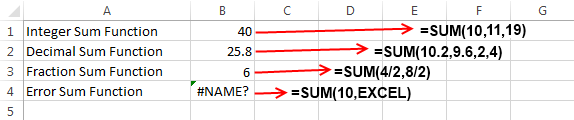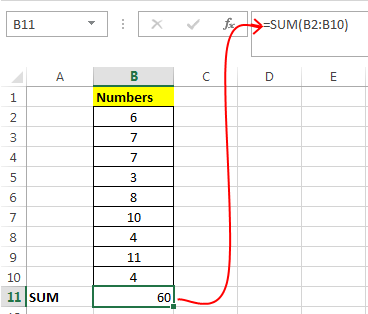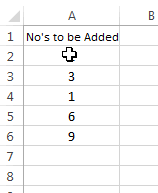# SUM Function in Excel – How to Use

SUM Function is a very popular and useful formula in Microsoft Excel. It is one of the most basic, widely used and easy to understand arithmetic functions in Excel. As the name suggests SUM Function in Excel performs the addition of numbers. Sum Function can accept numbers both as individual arguments and also as a complete range of cells.

### How Excel Defines SUM Function:

Microsoft Excel defines SUM as a formula that “Adds all the numbers in a range of cells”. This definition clearly points that Sum function has a job to add numbers and the arguments can be supplied using combinations of both numbers and range of cells.

### Syntax of SUM Function in Excel:

Sum function has two syntaxes and hence they can be written in two different ways:

`=SUM( num1, num2, ... num_n )`

Here ‘num1’, ‘num2’ and ‘num_n’ specifies numbers which you wish to add.

OR

`=SUM ( CellRange1, CellRange2, ... CellRange_n )`

Here ‘CellRange1’, ‘CellRange2’, ‘CellRange_n’ specifies multiple cell ranges (containing numbers) which are to be added.

### Some Important Facts about SUM Formula:

• Sum function can also do the addition of decimal numbers and fractions.
• If you are using SUM formula as =SUM( num1, num2, … num_n ) and in place of ‘num’ you have entered a non-numeric content then Sum function will throw an #Name? error.
• But if you are using SUM Function as =SUM ( CellRange1, CellRange2, … CellRange_n ) and if any of the cell range contains a non-numeric content then Sum function will ignore this value.
• Sum function is not a dynamic type of function. This means that if you have applied a Sum formula on a range of cells and then you filter out some values then the output of Sum function won’t change as it doesn’t change its result according the current values in the filter. It is better to use Subtotal function in such cases.

### Example of SUM Formula:In the above example I have applied four types of SUM functions and below I will explain them one by one.

1. In the first example I have used a function `=SUM(10,11,19)`. This Sum function simply adds-up all the values i.e. 10, 11 and 19 and hence the result is 40.

2. In the second example I have used a function `=SUM(10.2,9.6,2,4)`. Simple mathematics can tell us that 10.2+9.6+2.4 is 25.8 and hence the output is 25.8.

3. In third example a fraction type Sum function is used `=SUM(4/2,8/2)`. As 4/2 results into 2 and 8/2 results into 4 and addition of both these gives the resultant 6.

4. In the fourth example Sum function is used with a non-numeric value `=SUM(10,EXCEL)` and this is the reason why it results into a #Name? error.5. In the above image I have used second type of SUM function [`=SUM(B2:B10)`] to which I have supplied a range of cells (B2:B10) as arguments. This sum function simply adds each element of the range and delivers the result as 60.

### Shortcut for SUM Formula:

Instead for applying sum formula in the normal way, you can also apply the SUM Formula using a shortcut.Simply select the range (containing your numbers to be added), then press “Alt +” key and the desired sum will be populated in the next cell.

### Using Mathematical Operators inside SUM Function:

Mathematical operators like (+,-, / and *) can also be used inside Sum functions. This means that a SUM function as `=SUM(2*4)` is valid and it will result into 8. Similarly, a SUM Function `=SUM(62-4)` is also valid and it results into 58.

Actually, this happens because Sum Function treats this whole set of numbers and operators as a single number. So, first of all it evaluates the result of this mathematical operation and as there is no other argument supplied to the function so it shows the result as it is.About Ankit Kaul

Ankit is the founder of Excel Trick. He is tech Geek who loves to sit in front of his square headed girlfriend (his PC) all day long. :D. Ankit has a strong passion for learning Microsoft Excel. His only aim is to turn you guys into 'Excel Geeks'.